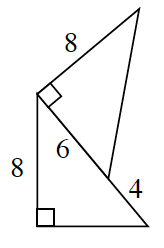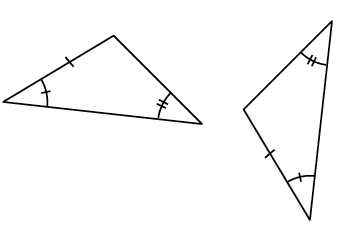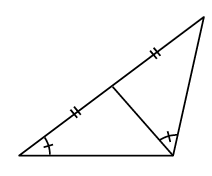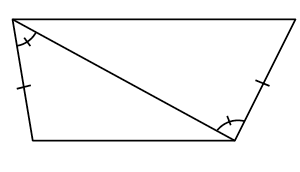### Home > CCG > Chapter 6 > Lesson 6.2.4 > Problem6-83

6-83.

Determine whether or not the triangles in each pair below are congruent. Justify your conclusion with a triangle congruency condition. Then choose one pair of congruent triangles and show your reasoning with a flowchart.

1.Find the lengths of the missing sides using the Pythagorean Theorem.

Congruent by $\text{HL}≅$, $\text{SSS}≅$, $\text{SAS}≅$.

1.What do the tick marks on the angles and sides mean?

1.Do the tick marks prove the triangles are congruent, or is more information needed?

They are not necessarily congruent. There is no such thing as $\text{SAS}≅$.

1.Does the fact that they share a side help prove they are congruent?